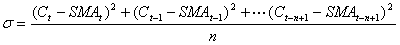Chart Pro online
User name (email):

 Recently used

 Forex

 Indices

# Standard Variance (SMV)

Standard variance (SMV) is statistical measure of volatility.

Standard variance is calculated by formula:Standard variance is calculated as square of standard deviation. Default value for parameter n is 21.Standard Variance (SMV)

Interpretation

Standard variance is not indicator which is used for creating buying and selling signals.

When standard variance readings are normal market is stable.

Example